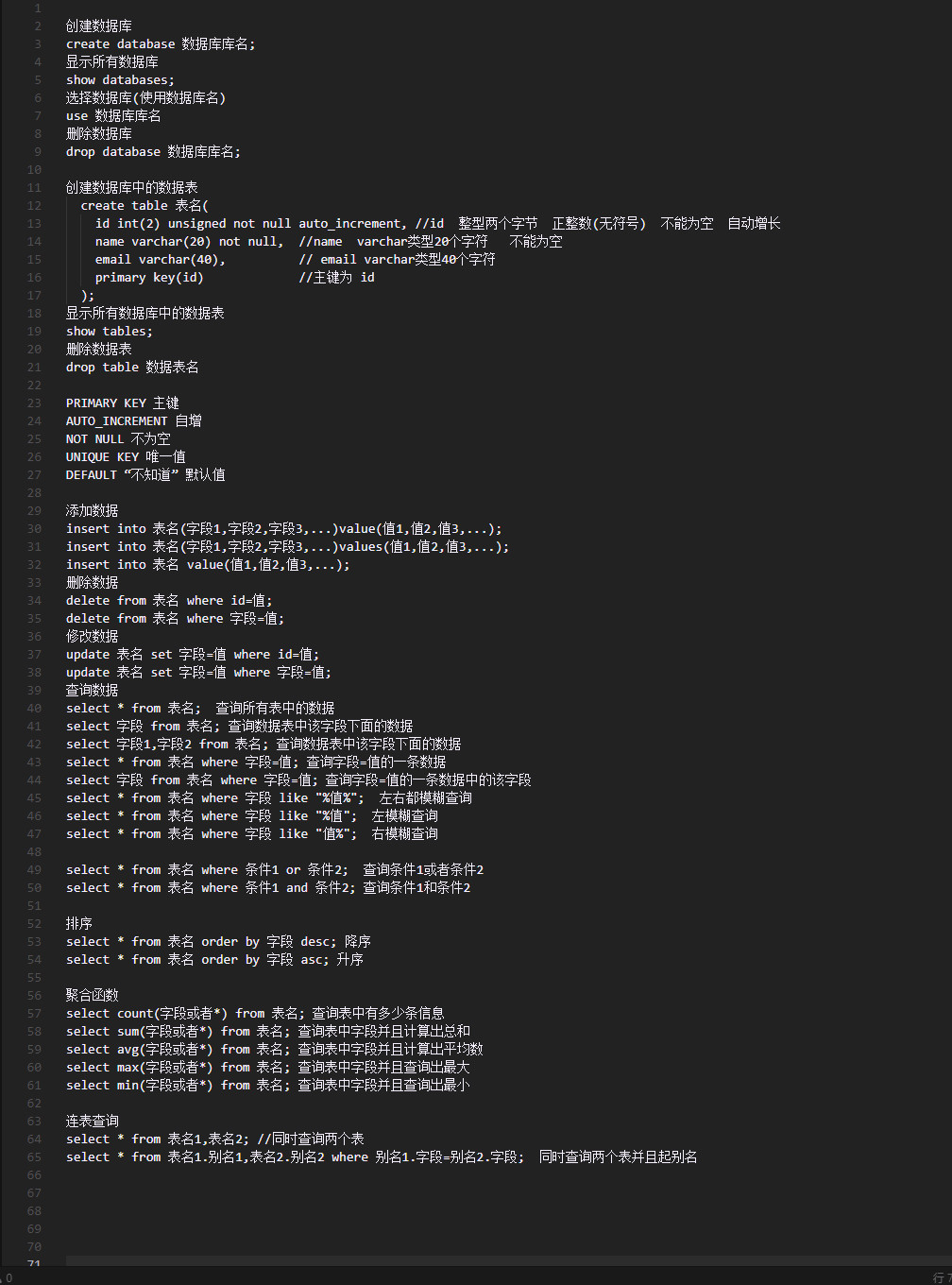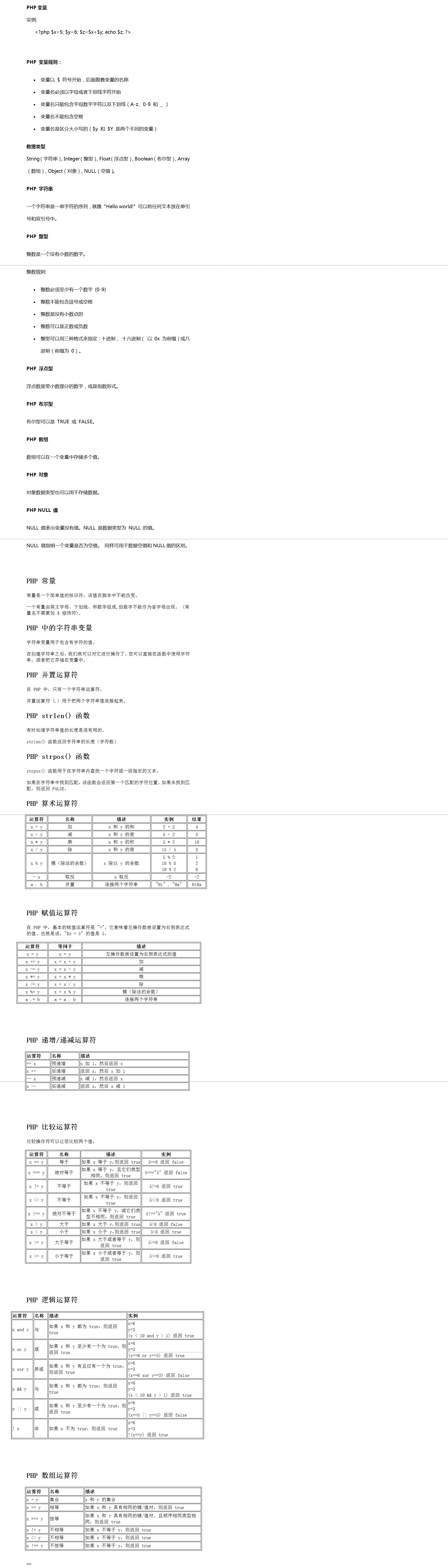PHP变量

``````<?php \$x=5; \$y=6; \$z=\$x+\$y; echo \$z; ?>
``````

PHP 变量规则：
• 变量以 \$ 符号开始，后面跟着变量的名称
• 变量名必须以字母或者下划线字符开始
• 变量名只能包含字母数字字符以及下划线（A-z、0-9 和 _ ）
• 变量名不能包含空格
• 变量名是区分大小写的（\$y 和 \$Y 是两个不同的变量）

String（字符串）, Integer（整型）, Float（浮点型）, Boolean（布尔型）, Array（数组）, Object（对象）, NULL（空值）。
PHP 字符串

PHP 整型

• 整数必须至少有一个数字 (0-9)
• 整数不能包含逗号或空格
• 整数是没有小数点的
• 整数可以是正数或负数
• 整型可以用三种格式来指定：十进制， 十六进制（ 以 0x 为前缀）或八进制（前缀为 0）。
PHP 浮点型

PHP 布尔型

PHP 数组

PHP 对象

PHP NULL 值
NULL 值表示变量没有值。NULL 是数据类型为 NULL 的值。
NULL 值指明一个变量是否为空值。 同样可用于数据空值和NULL值的区别。

PHP 常量

PHP 中的字符串变量

PHP 并置运算符

PHP strlen() 函数

strlen() 函数返回字符串的长度（字符数）
PHP strpos() 函数
strpos() 函数用于在字符串内查找一个字符或一段指定的文本。

PHP 算术运算符

x + y 加 x 和 y 的和 2 + 2 4
x - y 减 x 和 y 的差 5 - 2 3
x y 乘 x 和 y 的积 5 2 10
x / y 除 x 和 y 的商 15 / 5 3
x % y 模（除法的余数） x 除以 y 的余数 5 % 2
10 % 8
10 % 2 1
2
0

• x 取反 x 取反 -2 -2
1. . b 并置 连接两个字符串 "Hi" . "Ha" HiHa

PHP 赋值运算符

x = y x = y 左操作数被设置为右侧表达式的值
x += y x = x + y 加
x -= y x = x - y 减
x = y x = x y 乘
x /= y x = x / y 除
x %= y x = x % y 模（除法的余数）
a .= b a = a . b 连接两个字符串

PHP 递增/递减运算符

++ x 预递增 x 加 1，然后返回 x
x ++ 后递增 返回 x，然后 x 加 1
-- x 预递减 x 减 1，然后返回 x
x -- 后递减 返回 x，然后 x 减 1

PHP 比较运算符

x == y 等于 如果 x 等于 y，则返回 true 5==8 返回 false
x === y 绝对等于 如果 x 等于 y，且它们类型相同，则返回 true 5==="5" 返回 false
x != y 不等于 如果 x 不等于 y，则返回 true 5!=8 返回 true
x <> y 不等于 如果 x 不等于 y，则返回 true 5<>8 返回 true
x !== y 绝对不等于 如果 x 不等于 y，或它们类型不相同，则返回 true 5!=="5" 返回 true
x > y 大于 如果 x 大于 y，则返回 true 5>8 返回 false
x < y 小于 如果 x 小于 y，则返回 true 5<8 返回 true
x >= y 大于等于 如果 x 大于或者等于 y，则返回 true 5>=8 返回 false
x <= y 小于等于 如果 x 小于或者等于 y，则返回 true 5<=8 返回 true

PHP 逻辑运算符

x and y 与 如果 x 和 y 都为 true，则返回 true x=6
y=3
(x < 10 and y > 1) 返回 true
x or y 或 如果 x 和 y 至少有一个为 true，则返回 true x=6
y=3
(x==6 or y==5) 返回 true
x xor y 异或 如果 x 和 y 有且仅有一个为 true，则返回 true x=6
y=3
(x==6 xor y==3) 返回 false
x && y 与 如果 x 和 y 都为 true，则返回 true x=6
y=3
(x < 10 && y > 1) 返回 true
x || y 或 如果 x 和 y 至少有一个为 true，则返回 true x=6
y=3
(x==5 || y==5) 返回 false
! x 非 如果 x 不为 true，则返回 true x=6
y=3
!(x==y) 返回 true

PHP 数组运算符

x + y 集合 x 和 y 的集合
x == y 相等 如果 x 和 y 具有相同的键/值对，则返回 true
x === y 恒等 如果 x 和 y 具有相同的键/值对，且顺序相同类型相同，则返回 true
x != y 不相等 如果 x 不等于 y，则返回 true
x <> y 不相等 如果 x 不等于 y，则返回 true
x !== y 不恒等 如果 x 不等于 y，则返回 true